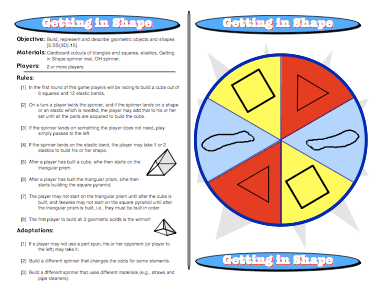# Getting in Shape: Geometry Math GamesThe Getting in Shape game gives kids the opportunity to build some models of 3-D geometric figures. Three simple pieces are all that are needed to make these models. A square, a triangle, and elastic bands (to connect the pieces) are used to create cubes, triangular prisms, and square pyramids. There is a luck factor of course! Each student has to spin the pieces he or she needs when they’re needed. After all, a triangle won’t help in building a cube. There are different ways to create the same figure as well. For example, with the cube, a student could spin enough squares to construct the cube or could connect different squares with bands at the appropriate spots.

The 3-D figures must be constructed in a specific order. The cube is constructed first, followed by the triangular prism, then followed by the square pyramid. By constructing these figures, students understand how they are built much more thoroughly than by simply studying a 2-D flat version of the figure that they view on a screen or text page.

For more advanced students, this game can also be a springboard to discuss simple probability. For example, suppose Katie needs a triangle to complete her figure. What is the probability that she’ll land on a triangle when she spins the spinner? It’s an interesting experiment to keep a chart of data that shows how many spins it took to create a particular figure. If the data is kept for all three figures for each player in the group, the class can draw conclusions about which figure required the most spins to complete.

Common Core Mathematical Standards
G-GMD isualize relationships between two-dimensional and three-dimensional objects.

Common Core Standards for Mathematical Practice
4. Model with Mathematics

This site uses Akismet to reduce spam. Learn how your comment data is processed.